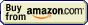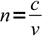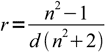# Refractometry## Refractometry » Refractive index

While it was not obvious to Snellius when he was researching the refraction, refractive index doesn't measure the refraction, but rather the relative light speed in both media involved.

As it was already explained on Snell's law page, Snellius has selected vacuum and assigned 1 to its intrinsic property responsible for refraction. We know now that it was a good idea - speed of light in vacuum is the maximum speed possible, so it is a perfect reference. Using this information we can now write a definition of a refractive index of a substance using light speeds:1

where c is light speed in vacuum, and v is a light speed in the substance (which usually is a function of wavelength). Such a refractive index is sometimes called the absolute refractive index, as opposed to relative refractive index given for a boundary between two media of which none is vacuum.

Note, that the same approach works perfectly well for any other waves travelling through any other media - for example sound, or waves on the water surface.

Absolute refractive index is usually higher than 1. Using cleverly designed metamaterials, physicists were able to prepare media in which refractive index is negative, but explanation of the way they work is not possible using simple approach based on the speed of light.

In most substances speed of light (and refractive index) doesn't depend on the direction of propagation and/or light polarization, but in some materials (like calcite) it does. In such materials we observe so called birefringence - light gets split into two polarized rays.

Refractive index is temperature dependent. However, combining refractive index and substance density it is possible to define a quantity that is temperature independent. It is called specific refraction:2

where r - specific refraction, d - density, n - refractive index

As refractive index depends on the wavelength, refractive index tables usually contain information about the wavelength used for measurement. In most cases values of refractive index are listed for yellow sodium light with wavelength of 589.3 nanometers.

This site is in construction. More to come. Page was last modified on March 17 2011, 00:00:00.